Chapter 2 National Income Accounting - Basic Concepts

Economics Class 12
Macroeconomics

#### GROSS INVESTMENT,DEPRECIATION AND NET INVESTMENT

Suppose I start a new business with Stock of Rs 5,00,000 and Machine of Rs 10,00,000

How much is my Gross Investment in Business

Gross investment = 5,00,000 + 10,00,000 = 15,00,000

Suppose after 1 year, Value of Machine is now worth Rs 8,00,000

What is Depreciation

Depreciation = 10,00,000 - 8,00,000 = 2,00,000

What is Value of my Investment Now

 Gross Investment 15,00,000 Less Depreciation 2,00,000 Net Investment 13,00,000

#### Gross Investment

It is the gross addition to capital stock of economy

Capital Stock means Fixed Assets + Unsold Stock

It is calculated before Depreciation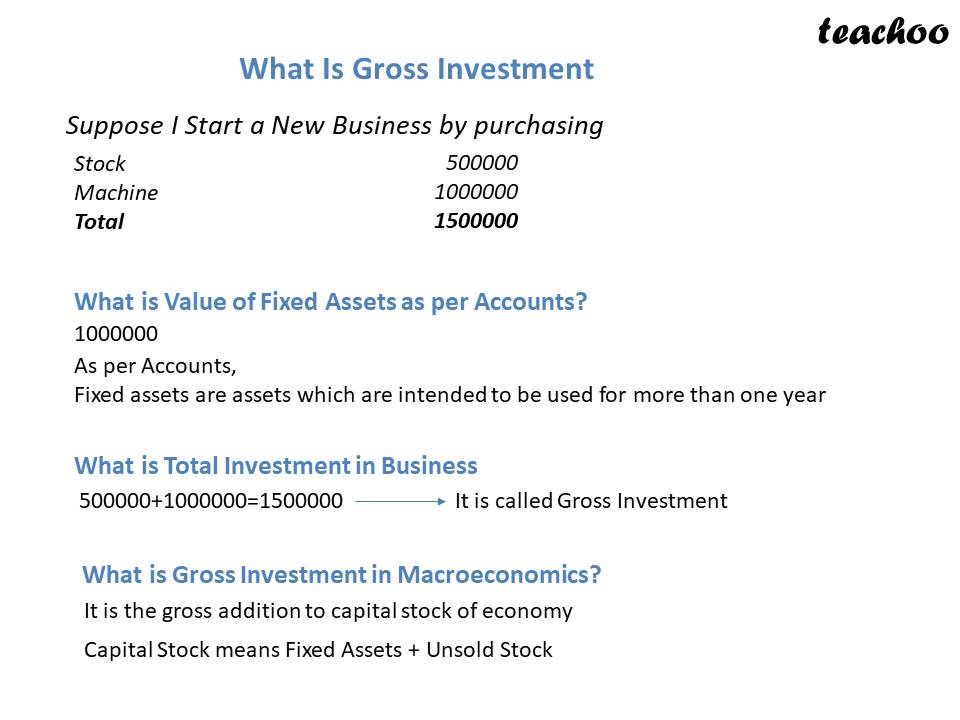#### Depreciation

It is the fall in the value of fixed assets due to Normal wear and tear, obsolescence and passage of time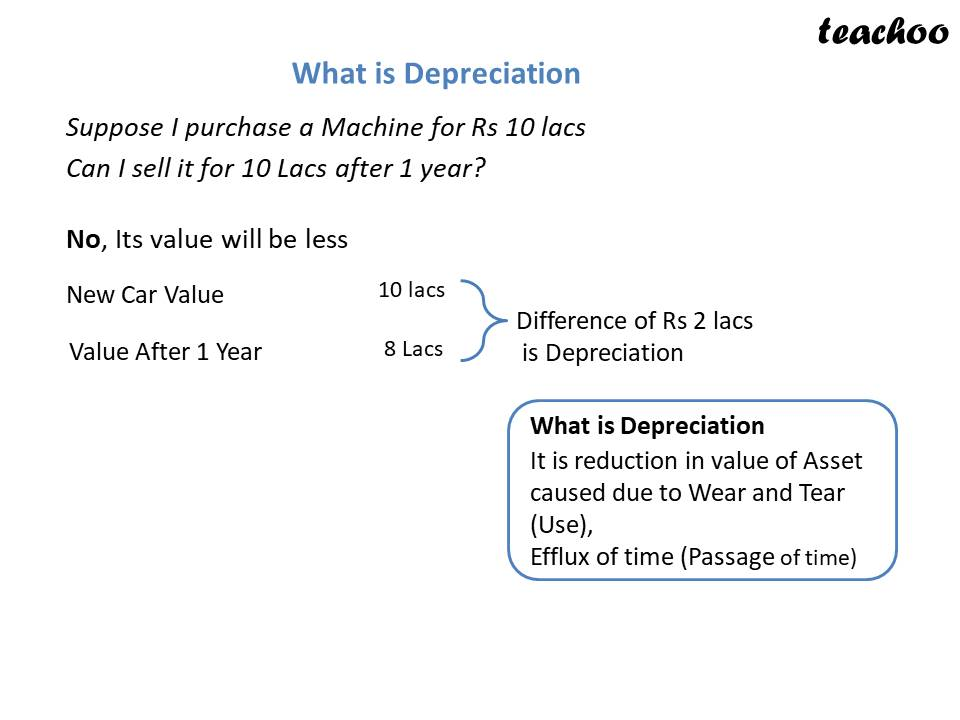#### Reason for Deprecation

Wear and Tear              On using Assets, it gets damaged

Obsolescence               Technology becomes outdated

Passage of Time           Some assets have limited life. They become outdated after this period

Hence Assets purchased today have to be replaced in future

To account for this expense, Depreciation is recorded in books

Example

Suppose I purchase a Mobile Phone for Rs 30,000

It is expected to last 3 years

After 3 years, I have to replace this phone with a new phone and spend Rs 30,000

So my cost of using phone for 3 years is Rs 30,000

Cost of using phone for 1 year = Rs 10,000

In this case, I will record Depreciation each year of Rs 10,000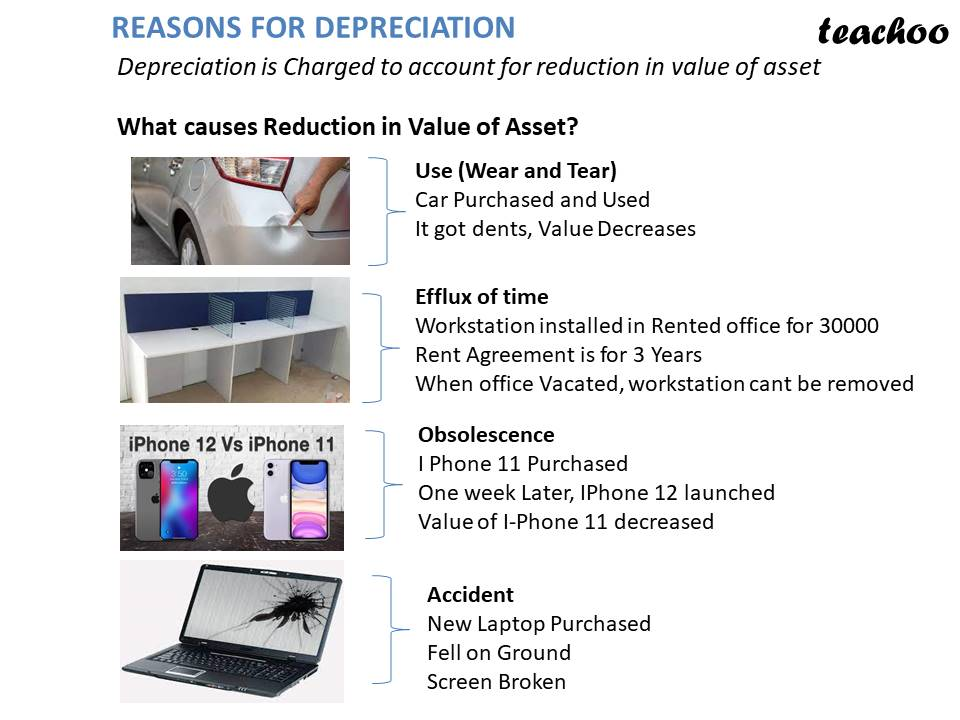#### What is Net Investment?

Net Investment = Gross Investment - Depreciation

It is Net addition to Capital Stock (Capital Goods) of an economy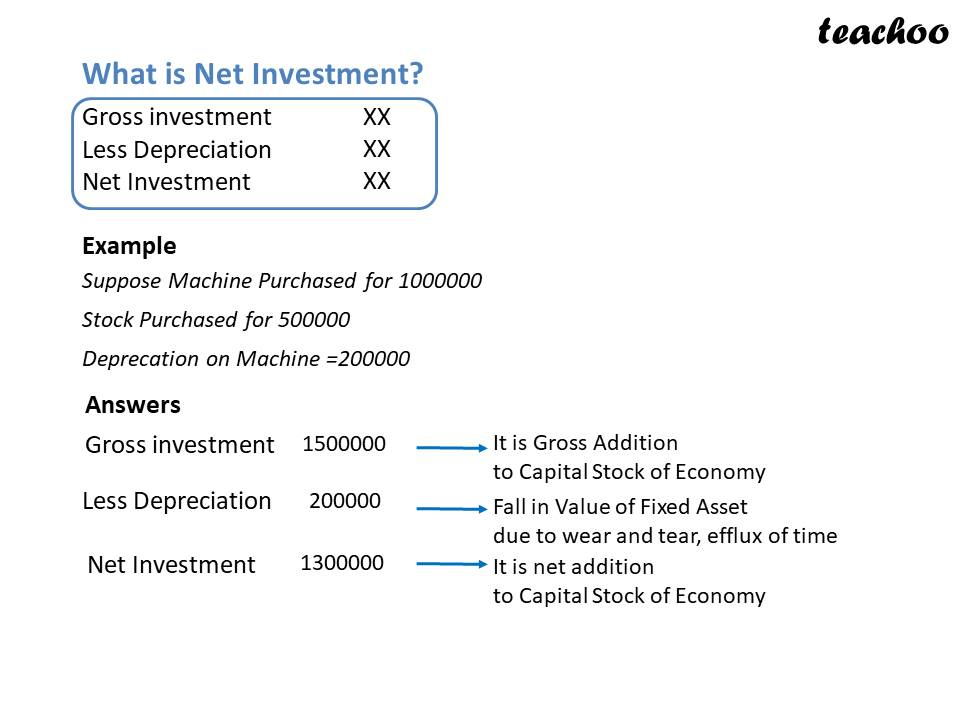#### Is Depreciation a Real Expenditure?

No, it is not actual expenditure

It is just an accounting concept

No real expenditure may be incurred, still Depreciation is recorded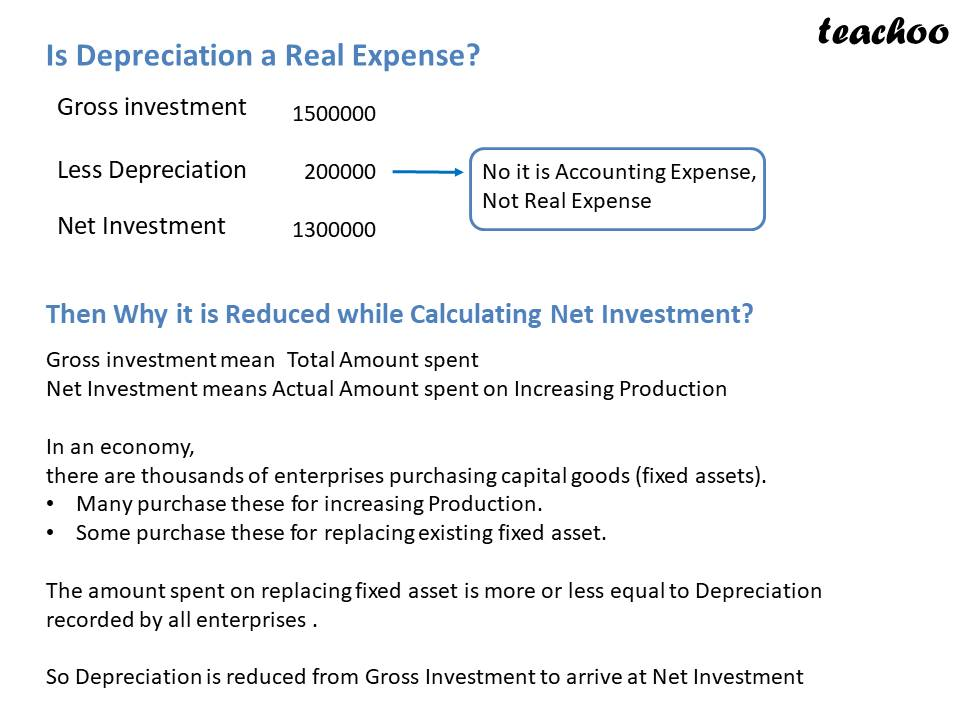#### If Depreciation is not a real expenditure, why is it reduced while calculating Net Investment?

Net Investment means Actual amount spent on increasing Production in an economy

In an economy, there are thousands of enterprises purchasing capital goods (fixed assets).

Many purchase these for increasing Production

Some purchase these for replacing existing fixed asset

The amount spent on replacing fixed asset is more or less equal to Depreciation recorded by all enterprises

Hence, Depreciation is reduced from Gross Investment to arrive at Net Investment

Example

Suppose there are 3 firms in Economy

They purchase machine as follows

A             100 -->This Purchase is for Increasing production

B             600 -->This Purchase is for Increasing production

C             200 -->This Purchase is for Replacing existing Machine

900

#### What is Gross Investment, Depreciation and Net Investment of Economy?

Gross Investment    900  (Total Investment on Capital Goods by all firms)

Depreciation            -200 --> It is equal to cost of Replacing Fixed Assets

Net Investment         700  (Actual Amount Spent on Increasing Production)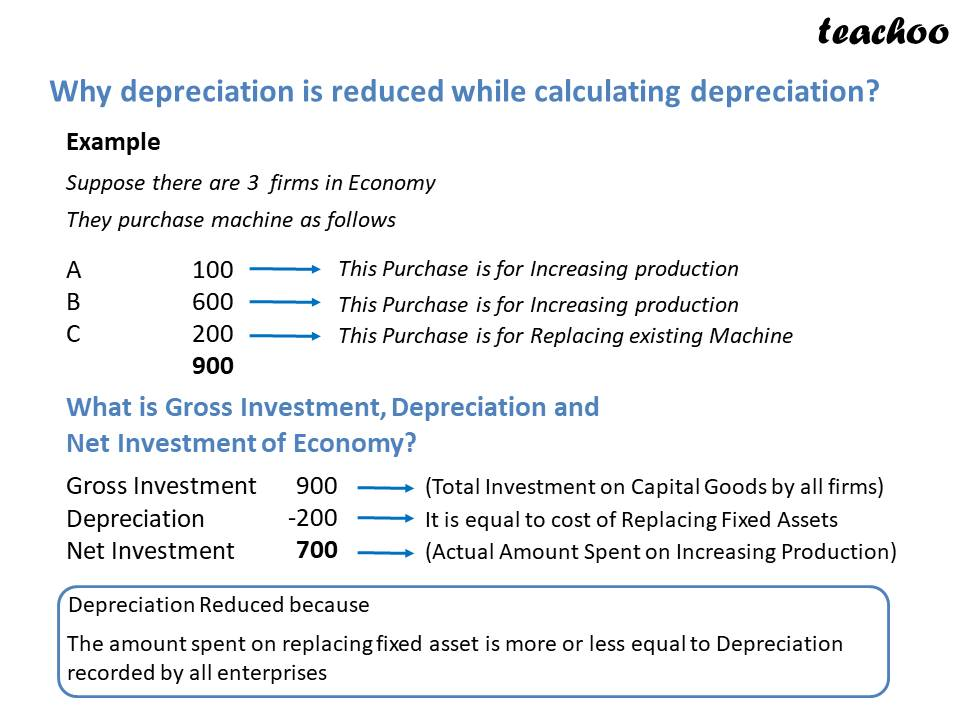## NCERT Questions

No questions in this part

## Other Books

### Question 1

When ___ is subtracted from gross investment, we get Net Investment.

### Question 2

State true or false with reasons.

1. Gross investment is equal to net investment.

### Question 3

Define depreciation.

### Question 4

'Gross investment is zero when net investment is zero.'

Defend or refute.

Learn in your speed, with individual attention - Teachoo Maths 1-on-1 Class

### Transcript

Gross Investment It is the gross addition to capital stock of economy Capital Stock means Fixed Assets + Unsold Stock It is calculated before Depreciation WHAT IS GROSS INVESTMENT Suppose I Start a New Business by Purchaing Stock MACHINE Total 500000 1000000 1500000 What is Value of Fixed Assets as per Accounts? 1000000 (Those assets which are intended to be used for more than one year are called Fixed Asset What is Value of Gross Investment in Business 500000+1000000=1500000 It is the gross addition to capital stock of economy Capital Stock means Fixed Assets + Unsold Stock Depreciation It is the fall in the value of fixed assets due to Normal wear and tear, obsolescence and passage of time What is Depreciation Suppose I purchase a Machine for Rs 10 lacs Can I sell it for 10 Lacs after 1 year? No Its value will be less New Car Purchased for 100000 Value After 1 Year 800000 Difference of Rs 200000 is Depreciation What is Depreciaiton It is reduction in value of Asset caused due to Wear and Tear (Use), Efflux of time (Passage of time) REASONS FOR DEPRECIATION What causes Reduction in Value of Asset (Why is Depeciation Charged) Use (Wear and Tear) Car Purchased and Used It got dents,Value Decreases Efflux of time Workstation installed in Rented office for 30000 Rent Agreement is for 3 Years When office Vacated,workstation cant be removed Obsolecense I Phone 11 Purchased One week Later,IPHone 12 launched Value of I Phone 12 decreased Accident New Laptop Purchased Fell on Ground Screen Broken What is Net Investment? Net Investment = Gross Investment - Depreciation It is Net addition to Capital Stock (Capital Goods) of an economy Is Depreciation a Real Expenditure? No, it is not actual expenditure It is just an accounting concept No real expenditure may be incurred, still Depreciation is recorded What is Net Investment? Gross investment Less Depreciaton Net Investment Example Suppose Machine Purchased for 1000000 Stock Purchased for 500000 Deprecition on Machine =200000 Gross investment Less Depreciaton Net Investment 1500000 200000 1300000 --> It is Gross Addition to Capital Stock of Eonomy --> Fall in Value of Fixed Asset --> It is net Addition to Capital Stock of Economy Is Depreciation Real Expense? Gross investment Less Depreciaton Net Investment 1500000 200000 1300000 --> No it is Accounting Expense,Not Real Expense ThenWhy it is Reduced while Calculatin Net Investment? Net Investmen means Actual Amount spent on Increasing Production note In an economy,there are thousands of enterprises purchasing capital goods (fixed assets). Many purchase these for increasing Production Some purchase these for replacing existing fixed asset The amount spent on replacing fixed asset is more or less equal to Depreciation recorded by all enterprises Hence,Depreciation is reduced from Gross Investment to arrive at Net Investment Example-Why Deprecation is Reduced while Calculating Deprecation Example Suppose there are 3 firms in Economy They purchase machine as follows 100 600 200 900 This Purchase is for Increasing production This Purchase is for Increasing production -->This Purchase is for Replacing existing Machine What is Gross Investment, Depreciation and Net Investment of Economy? Gross Investment Depreciation Net Investment 900 (Total Investment on Capital Goods by all firms) -200 It is equal to cost of Replacing Fixed Assets 700 (Actual Amount Spent on Increasing Production) Deprecation Reduced because The amount spent on replacing fixed asset is more or less equal to Depreciation recorded by all enterprises If Depreciation is not a real expenditure, why is it reduced while calculating Net Investment? Net Investment means Actual amount spent on increasing Production in an economy In an economy, there are thousands of enterprises purchasing capital goods (fixed assets). Many purchase these for increasing Production Some purchase these for replacing existing fixed asset The amount spent on replacing fixed asset is more or less equal to Depreciation recorded by all enterprises Hence, Depreciation is reduced from Gross Investment to arrive at Net Investment NCERT Questions No questions in this part Other Books Q1 When ___ is subtracted from gross investment, we get Net Investment. -a- depreciation -ea- Q2 State true or false with reasons. 1. Gross investment is equal to net investment. -a- True It is possible if depreciation is equal to 0. -ea- Q3 Define depreciation. -a- The fall in the value of fixed assets due to Normal wear and tear, obsolescence and passage of time is known as Depreciation. -ea- Q4 'Gross investment is zero when net investment is zero.' Defend or refute. -a- This statement is refuted because gross investment is not 0 when net investment is 0. Net Investment = Gross Investment - Depreciation (Taking Net Investment = 0) 0 = Gross Investment - Depreciation Depreciation = Gross Investment From this equation, we can see Gross Investment = Depreciation when Net Investment is 0 -ea-